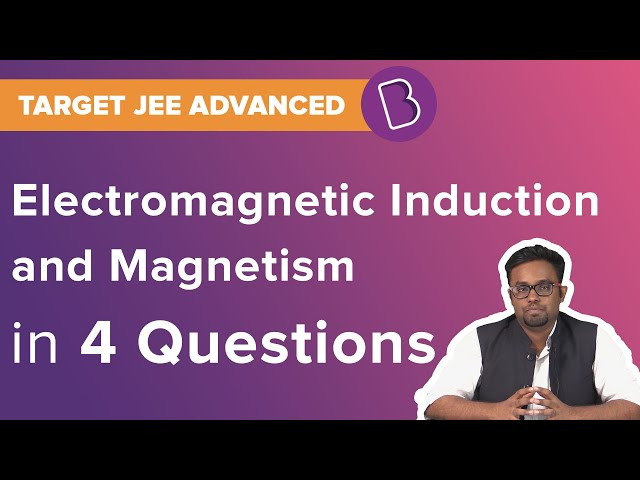# Electromagnetic Induction Questions

The process of electromagnetic induction generates a voltage or Electromotive Force (EMF) across the electrical conductor due to a changing magnetic field. Electromagnetic induction is generally referred to as induction.

In 1831 electromagnetic induction was discovered by Michael Faraday, and later James Clerk Maxwell mathematically described it as Faraday’s law of induction. The formula gives electromagnetic induction:

e = N × dΦ / dt

Where,

e – induced voltage (in volts)

N – number of turns in the coil

Φ – magnetic flux (in Webbers)

t -time (in seconds)

The induction of an electromotive force by the motion of a conductor across a magnetic field or by a change in magnetic flux in a magnetic field is known as Electromagnetic Induction. This phenomenon takes place when a conductor is set in a moving magnetic field or when a conductor is always moving in a stationary magnetic field.

The concept of electromagnetic induction is used in generators, inductors, motors, and transformers. The magnetic flow meter is based on this concept too.

The first and second laws of electromagnetic induction are:

First law: Whenever a conductor is placed in a varying magnetic field, EMF induces, and this EMF is called an induced EMF. If the conductor is a closed circuit then the induced current flows through it.

Second law: The magnitude of the induced EMF is equal to the rate of change of flux linkages.

The amount of voltage induced in a coil is proportional to the number of turns of the coil and the rate of changing the magnetic field.

Lenz’s law of electromagnetic induction states that, when an emf induces according to Faraday’s law, the polarity (direction) of that induced emf is such that it opposes the cause of its production.

According to Lenz’s law

E = -N (dΦ/ dt) (volts)

A step-down transformer is the one in which the voltage is higher in the primary than the secondary voltage.

Important Electromagnetic Induction Questions with Answer

1. The direction of the induced field can be predicted by____

1. Kepler’s law
2. Newton’s law
3. Lenz’s law

Explanation: Lenz’s law states that the direction of the electric current induced in a conductor by a changing magnetic field is such that the magnetic field created by the induced current opposes changes in the initial magnetic field.

2. Fill in the blanks: Electric generator converts electrical energy into____

1. Chemical energy
2. Thermal energy
3. Electrical energy
4. Solar energy

3. The phenomenon called electromagnetic induction was first investigated by

1. Newton
2. Kepler
4. Galileo

Explanation: Electromagnetic Induction was discovered by Michael Faraday in 1831

4. Magnetic flux is represented as

1. $$\begin{array}{l}\Theta\end{array}$$
2. $$\begin{array}{l}\epsilon\end{array}$$
3. $$\begin{array}{l}\alpha\end{array}$$
4. $$\begin{array}{l}\phi\end{array}$$

$$\begin{array}{l}\phi\end{array}$$

5. Factors that affect the voltage generation in Faraday’s experiment is

1. Number of Coils
2. Changing Magnetic Field
3. Changing Environment
4. Option a) and b)

Explanation: Changing magnetic field and the number of coils affects the voltage production in Faraday’s experiment.

6. Define magnetic flux.

The number of magnetic field lines passing through a given closed surface gives magnetic flux.

7. Lenz’s law is derived from ____.

1. Kepler’s law
2. Newton’s law
3. Coulomb’s Law

8. State true or false: Eddy currents flow in closed loops in planes perpendicular to the magnetic field.

1. TRUE
2. FALSE

9. The SI unit of magnetic flux is

1. Kilogram
2. Weber
3. Second
4. Candela

Explanation: The SI unit of magnetic flux is Weber.

10. The induced voltage is found according to Faraday’s law using the formula____

1. e = N × dΦdt
2. e = N × dΦ/dt
3. e = N + dΦdt
4. e = N – dΦdt

Answer: a) e = N × dΦdt

## Practice Questions